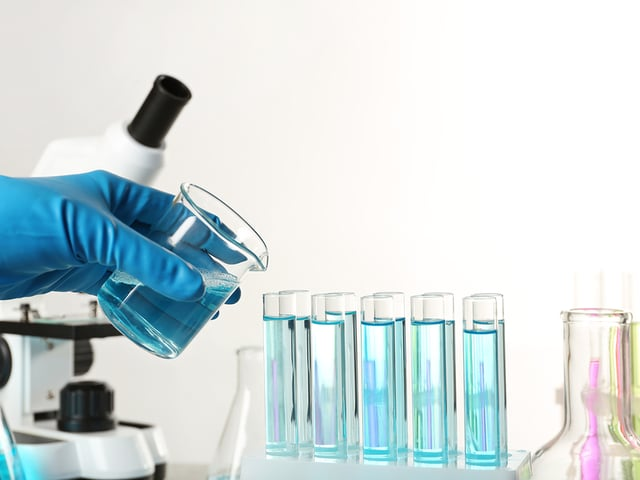# Formulas You’ll Need to Know for the HESI® Chemistry Test

If you’re preparing for the HESI® test, you probably know that the HESI® Chemistry section is really important. After all, chemistry is going to be a big part of your nursing career. Chemistry is special in the sense that it combines theoretical and mathematical knowledge.

## How Do I Get Ready for This?

You can find the theoretical knowledge you need in our free study guide for the HESI® Chemistry Test.

And for the mathematical knowledge part, we have something special for you.

The following chart will help you to know which formulas you need to succeed on the HESI® Chemistry test. You won’t be able to use it during the test, but it is a valuable tool to help you prepare. Don’t forget to use it to solve our free practice questions for the HESI® Chemistry Test.

$\begin{array}{|c|c|c|} \hline \textbf{Category} & \textbf{Formula} & \textbf{Symbols} \\ \hline & & d = \text{ density } (g/cm^3) \\ \text{Chemistry} & d = \dfrac{m}{v} & m = \text{ mass } (g) \\ & & v = \text{ volume } (cm^3)\\ \hline & & A = \text{ Mass Number } \\ \text{Chemistry} & ^{A}_{Z}X & Z = \text{ Atomic Number } = \text{ Number of Protons } \\ & & X = \text{ Atomic Symbol } \\ \hline & & A = \text{ Mass Number } \\ \text{Chemistry} & A = Z + N & Z = \text{ Atomic Number } = \text{ Number of Protons } \\ & & N = \text{ Number of Neutrons } \\ \hline & & n = \text{ number of moles } \\ \text{Chemistry} & n \cdot N_a = a & N_a = \text{ Avogadro's Number } = 6.022 \cdot 10^{23} (atoms/mole)\\ & & a = \text{ number of atoms } \\ \hline & & M = \text { molar mass } (g/mole) \\ \text{Chemistry} & M = \dfrac{m}{n} & m = \text{ mass } (g)\\ & & n = \text{ number of moles }\\ \hline \text{Chemistry} & m_r = m_p & m_r = \text{ total mass of reactants } (g) \\ & & m_p = \text{ total mass of products } (g) \\ \hline \text{Chemistry} & pH = - \log[H_3O^+] & pH = \text{ decimal cologarithm of Hydrogen } \\ & & [H_3O^+] = \text{ Hydronium conc. } (mole/L) \\ \hline \text{Chemistry} & 14 = pH + pOH & pH = \text{ decimal cologarithm of Hydrogen } \\ & & pOH = \text{ decimal cologarithm of Hydroxide } \\ \hline \end{array}$